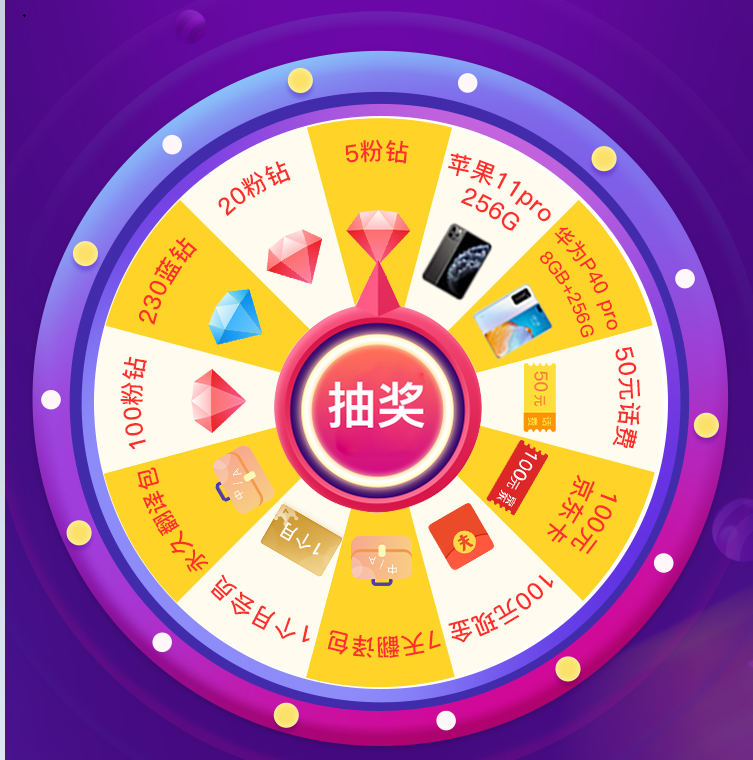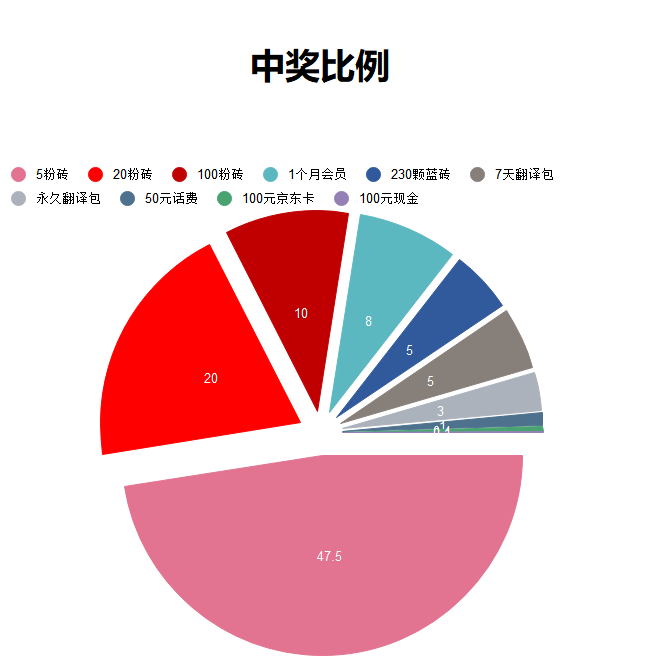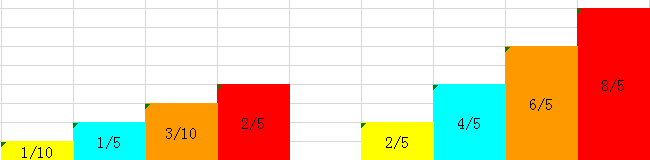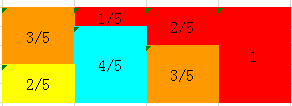# java版抽奖算法奖品名称 奖品类型 奖品中将概率% 100元现金红包 实物 0.1 100元京东充值卡 实物 0.4 50元话费 虚拟物品 1 永久翻译包 虚拟物品 3 7天翻译包 虚拟物品 5 230颗蓝钻 虚拟物品 5 1个月会员 虚拟物品 8 100粉钻 虚拟物品 10 20粉钻 虚拟物品 20 5粉钻 虚拟物品 47.5public class LottoRsa {
private static final List<Prize> prizePool=new ArrayList<Prize>() {
{
this.add(new Prize("100元现金"));
this.add(new Prize("100元京东卡"));
this.add(new Prize("50元话费"));
this.add(new Prize("永久翻译包"));
this.add(new Prize("7天翻译包"));
this.add(new Prize("230颗蓝砖"));
this.add(new Prize("1个月会员"));
this.add(new Prize("100粉砖"));
this.add(new Prize("20粉砖"));
this.add(new Prize("5粉砖"));
}
};

public static  Prize getPrize() {
int randomInt=RandomUtil.randomInt(100);
int randomFloat=RandomUtil.randomInt(10);

Float probability=Float.valueOf(randomInt+"."+randomFloat);

if(probability>=0&&probability<=0.1) {
return prizePool.get(0);
}
if(probability>=0.1&&probability<=0.5) {
return prizePool.get(1);
}
if(probability>=0.5&&probability<=1.5) {
return prizePool.get(2);
}
if(probability>=1.5&&probability<=4.5) {
return prizePool.get(3);
}
if(probability>=4.5&&probability<=9.5) {
return prizePool.get(4);
}
if(probability>=9.5&&probability<=14.5) {
return prizePool.get(5);
}
if(probability>=14.5&&probability<=22.5) {
return prizePool.get(6);
}
if(probability>=22.5&&probability<=32.5) {
return prizePool.get(7);
}
if(probability>=32.5&&probability<=52.5) {
return prizePool.get(8);
}
return prizePool.get(9);
}
public static void main(String[] args) {
for(int i=0;i<100;i++) {
System.out.println(getPrize());
}
}
}


 T 1 2 3 4 PDF 0.1 0.2 0.3 0.4 CDF 0.1 0.3 0.6 1T 1 2 3 4 PDF 0.1 0.2 0.3 0.4 Alias 3 4 4 NULL

public final class AliasMethod {
/* The random number generator used to sample from the distribution. */
private final Random random;

/* The probability and alias tables. */
private final int[] alias;
private final double[] probability;

/**
* Constructs a new AliasMethod to sample from a discrete distribution and
* hand back outcomes based on the probability distribution.
* <p/>
* Given as input a list of probabilities corresponding to outcomes 0, 1,
* ..., n - 1, this constructor creates the probability and alias tables
* needed to efficiently sample from this distribution.
*
* @param probabilities The list of probabilities.
*/
public AliasMethod(List<Double> probabilities) {
this(probabilities, new Random());
}

/**
* Constructs a new AliasMethod to sample from a discrete distribution and
* hand back outcomes based on the probability distribution.
* <p/>
* Given as input a list of probabilities corresponding to outcomes 0, 1,
* ..., n - 1, along with the random number generator that should be used
* as the underlying generator, this constructor creates the probability
* and alias tables needed to efficiently sample from this distribution.
*
* @param probabilities The list of probabilities.
* @param random        The random number generator
*/
public AliasMethod(List<Double> probabilities, Random random) {
/* Begin by doing basic structural checks on the inputs. */
if (probabilities == null || random == null)
throw new NullPointerException();
if (probabilities.size() == 0)
throw new IllegalArgumentException("Probability vector must be nonempty.");

/* Allocate space for the probability and alias tables. */
probability = new double[probabilities.size()];
alias = new int[probabilities.size()];

/* Store the underlying generator. */
this.random = random;

/* Compute the average probability and cache it for later use. */
final double average = 1.0 / probabilities.size();

/* Make a copy of the probabilities list, since we will be making
* changes to it.
*/
probabilities = new ArrayList<Double>(probabilities);

/* Create two stacks to act as worklists as we populate the tables. */
Deque<Integer> small = new ArrayDeque<Integer>();
Deque<Integer> large = new ArrayDeque<Integer>();

/* Populate the stacks with the input probabilities. */
for (int i = 0; i < probabilities.size(); ++i) {
/* If the probability is below the average probability, then we add
* it to the small list; otherwise we add it to the large list.
*/
if (probabilities.get(i) >= average)
large.add(i);
else
small.add(i);
}

/* As a note: in the mathematical specification of the algorithm, we
* will always exhaust the small list before the big list.  However,
* due to floating point inaccuracies, this is not necessarily true.
* Consequently, this inner loop (which tries to pair small and large
* elements) will have to check that both lists aren't empty.
*/
while (!small.isEmpty() && !large.isEmpty()) {
/* Get the index of the small and the large probabilities. */
int less = small.removeLast();
int more = large.removeLast();

/* These probabilities have not yet been scaled up to be such that
* 1/n is given weight 1.0.  We do this here instead.
*/
probability[less] = probabilities.get(less) * probabilities.size();
alias[less] = more;

/* Decrease the probability of the larger one by the appropriate
* amount.
*/
probabilities.set(more,
(probabilities.get(more) + probabilities.get(less)) - average);

/* If the new probability is less than the average, add it into the
* small list; otherwise add it to the large list.
*/
if (probabilities.get(more) >= 1.0 / probabilities.size())
large.add(more);
else
small.add(more);
}

/* At this point, everything is in one list, which means that the
* remaining probabilities should all be 1/n.  Based on this, set them
* appropriately.  Due to numerical issues, we can't be sure which
* stack will hold the entries, so we empty both.
*/
while (!small.isEmpty())
probability[small.removeLast()] = 1.0;
while (!large.isEmpty())
probability[large.removeLast()] = 1.0;
}

/**
* Samples a value from the underlying distribution.
*
* @return A random value sampled from the underlying distribution.
*/
public int next() {
/* Generate a fair die roll to determine which column to inspect. */
int column = random.nextInt(probability.length);

/* Generate a biased coin toss to determine which option to pick. */
boolean coinToss = random.nextDouble() < probability[column];

/* Based on the outcome, return either the column or its alias. */
/* Log.i("1234","column="+column);
Log.i("1234","coinToss="+coinToss);
Log.i("1234","alias[column]="+coinToss);*/
return coinToss ? column : alias[column];
}

public static void main(String[] args) {
TreeMap<String, Double> map = new TreeMap<String, Double>();
map.put("苹果11pro 256G", 0.0);
map.put("华为P40 pro", 0.0);
map.put("100元现金", 0.001);
map.put("100元京东卡", 0.004);
map.put("50元话费", 0.01);
map.put("永久翻译包",0.03);
map.put("7天翻译包", 0.05);
map.put("230颗蓝砖", 0.05);
map.put("一个月会员", 0.08);
map.put("100粉砖", 0.1);
map.put("20粉砖",0.2);
map.put("5粉砖",0.475);
List<Double> list = new ArrayList<Double>(map.values());
List<String> gifts = new ArrayList<String>(map.keySet());

AliasMethod method = new AliasMethod(list);

Map<String, AtomicInteger> resultMap = new HashMap<String, AtomicInteger>();

for (int i = 0; i < 100000; i++) {
int index = method.next();
String key = gifts.get(index);
if (!resultMap.containsKey(key)) {
resultMap.put(key, new AtomicInteger());
}
resultMap.get(key).incrementAndGet();
}
for (String key : resultMap.keySet()) {
System.out.println(key + "==" + resultMap.get(key));
}

}
}

5粉砖==47440

100元现金==111
230颗蓝砖==4988
100粉砖==10075
50元话费==952

20粉砖==20089
7天翻译包==4979

03-1904-09
10-31
09-22
02-12294
03-264167
12-20
07-243万+
06-021629
07-05
09-27
12-261万+
01-23810
12-06189
09-197140
01-07452
01-135725
©️2020 CSDN 皮肤主题: 成长之路 设计师:Amelia_0503